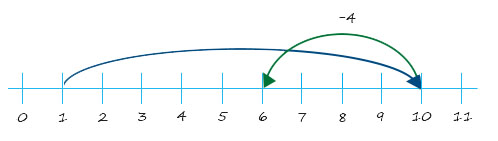# Using a number line for subtraction

Number line showing 10 – 4 = 6:Figure 4

Once students are used to using number lines for basic addition and subtraction with positive numbers then the line can be naturally extended to negative numbers.

Number lines are also easily extendable to two- or three-digit numbers.

Using a number line for adding on

3 Ways to demonstrate grouping and decomposition